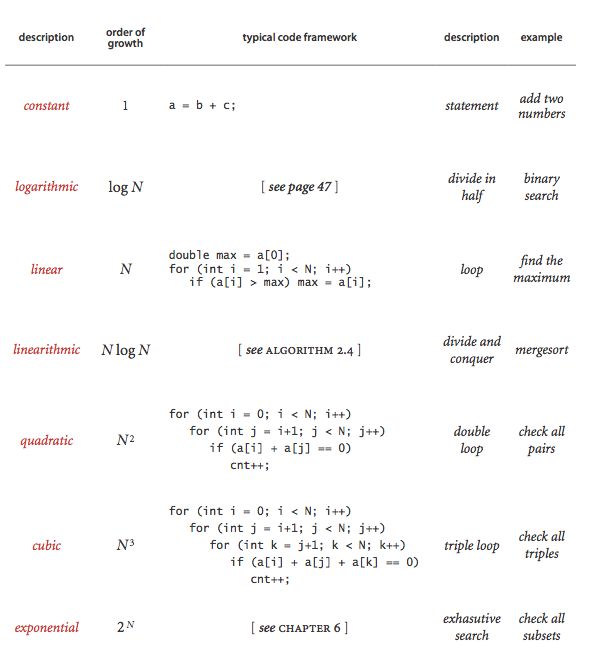# 算法分析

Wafer Li ... 2017-02-04 《Algorithm 第四版》笔记
• Algorithm
• 读书笔记

## # 1. 观察

• 运行程序，然后使用时间计算器来计算时间的使用。
• 使用大数据来猜测和验证数学模型

## # 2. 时间模型

• 每个操作的所需时间
• 每个操作使用的频率

### # 2.2 内循环

• 内循环指的是程序执行时，最频繁的操作
• 程序的时间复杂度一般都取决于内循环

### # 2.4 确定成本模型的步骤

1. 确定输入模型和问题规模
2. 确定内循环
3. 根据内循环来确定成本模型
4. 根据输入模型，来确定操作的频率和次数

### # 2.5 增长级别的分类1. 常数级别

大多数的 Java 基本操作 都是常数级别的。

2. 对数级别

比常数级别稍慢，例如 二分查找

3. 线性级别

单独的 for 循环

4. 线性对数级别

例如归并排序和快速排序

5. 平方级别

两个嵌套的 for 循环，例如选择排序，插入排序，冒泡排序都是平方级别的

6. 立方级别

三个嵌套的 for 循环是立方级别的，例如 3-sum 的暴力解法

7. 指数级别

非常慢，尽量避免去使用这种级别的算法

### # 2.6 设计更快的算法

3-sum 问题：即给出一个数和给定的数据集合，在数据集合中寻

• 2-sum 问题

2-sum 问题即为找到所有的数对，它们的和为 $0$，我们注意到，当 $A + B = 0$ 成立时，说明，两个数互为相反数，即 $A = -B$ 所以我们可以采取 二分查找 的方法来查找数据的相反数，从而查找到数对。

这样，我们就将时间复杂度由 平方级别($O(N^2)$) 减少到了 线性对数级别($O(NlogN)$)

注意，如果二分查找返回的 $j$$0$$i$ 之间，那么说明我们查找到了重复数据，（由于我们是对整个数组进行遍历，所以数据就会出现重复）应该不增加计数。

• 3-sum 问题

和 2-sum 问题一样，当 $-(a[i] + a[j])$ 在数组中（不是 $a[i]$ 也不是 $a[j]$ ）时，整数对 $(a[i], a[j])$ 是三元组的一部分。

由此，通过分治策略和使用 二分查找，我们将 $N^3$ 级别的问题降低到了 $N^2logN$ 级别。

### # 2.7 根据增长数量级做出的预测

| Describe | Function | Modulus is 2 | Modulus is 10 | Handle the 10N | Handle 10N in 10 times faster| |--------|------|------|-------|-----|--mathjax: true ---| | Linear| $N$|2|10| a day| couples of hours| | Linearrithmic|$NlogN$|2|10|a day | couples of hours| | Quadratic| $N^2$| 4| 100| a few weeks| a day| | Cubic| $N^3$ | 8 | 1000 | a few months | couples of weeks| | Exponential| $2^N$ | $2^N$ | $2^{10N}$ | forever | forever

### # 2.8 注意事项

1. 大常数

一般来说，我们认为 $2N^2 + cN$$\sim 2N^2$ 的，但是当 $c$$10^3$，或者 $10^6$ 的时候，这种估计是不正确的。

2. 非决定性的内循环

由于错误的内循环，导致了错误的成本模型，从而导致了错误的分析结果

3. 指令时间

对于当今的现代计算机，由于缓存技术的使用，使得访问大数组的非相邻元素所需的时间可能会很长

4. 系统因素

当你在运行算法分析程序的时候，可能你的电脑并不只是在运行这一程序，同时还运行着其他程序，这样的话就有可能导致算法分析的结果的不正确。

5. 不分伯仲

比较执行相同任务的程序时，通常会出现一个情形下这个算法比较好，另一个情形下另一个算法比较好的情况。

6. 对于输入的强烈依赖

在 3-sum 问题中，如果我们将问题改成 是否存在和为0的三个数？ 如果第一组的三个数都是 0，那么时间复杂度为常数级别，如果输入中不存在这样的数，则时间复杂度为 立方级别

在这种情况下，我们通常使用 $T_w$ （最坏情况的时间复杂度） 和 $T_{av}$ （平均时间复杂度） 来表示这种情况

7. 多个问题参量

成本模型并不总是单因素函数，也有可能是多因素函数。例如使用二分查找来进行白名单问题分析时，时间复杂度和 $MlogN$ 成正比

其中白名单有 $N$ 个整数，输入中有 $M$ 个整数

## # 3. 内存模型

### # 3.1 对象

• Integer
• 一共需要 24 byte
• 16 bytes 为对象本身的开销
• 4 bytes 的 int
• 对象的引用，一般为 内存地址，使用 8 byte

### # 3.2 链表

• Node(inner class)
• 一共 40 byte
• 16 bytes 为对象的开销
• 2 * 8 bytes 的引用（Node 中有两个引用）
• 当作为内部类时，需要一个额外的指向外围类的引用
• 数据的开销

### # 3.3 数组

• 数组
• 24 bytes 的头信息
• 16 bytes 对象开销
• 4 bytes 填补开销

### # 3.4 String

• 40 bytes
• Object cost, 16 bytes
• reference, 8 bytes
• 3 int, 12 bytes
• offset
• counter
• hash

$\Delta$ The subString

## # 4. 展望

• 不成熟的优化是万恶之源

Premature optimization is the root of all evil

• 如果运行时间已经足够快了，那么对运行时间的改进就不值得了

不值得花费 2 倍的开发时间来提高 10 % 的性能

• 但是我们当我们处理大型问题的时候，我们的确需要好的算法，好的算法在大规模问题中能带来巨大的收益